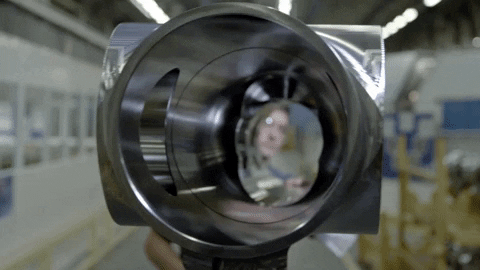top of page
Search
• aman bhati

# What Is Electroplating?

Updated: Feb 15, 2022## Definition

Electroplating is a process that uses an electric current to reduce dissolved metal cations so that they form a thin coherent metal coating on an electrode.

## Chemical reaction

Electroplating is the deposition process of a metallic film onto a seed metal layer, which works as a cathode (negative electrode) in an aqueous solution (bath) of a salt of the metal to be deposited. The electrochemical reaction between the metal M and its ions in the aqueous solution can be represented in simple formulae as below. Mz + (aq) + z e− → M(s)M(s) → Mz + (aq) + z e−

where Mz + is the metal ion and z is the number of electrons involved in the reaction. The amount of metal deposited is defined by the current that goes through to the electrode. Fig. 2.14 shows an example of an electrolytic cell used to electroplate copper. The seed layer is often a thin film deposited on a silicon or glass wafer, as shown in the figure.The chemical reaction of copper, for example, is presented in the equation below.

Cathode: Cu2 + (aq) + 2e− → Cu(s)

Anode: Cu(s) → Cu2 + (aq) + 2e−

References